# Consider the scatterplot of y versus x in Output B.6 above. a. Which power transformations on y should be considered to straighten the scatterplot? b. Which power transformations on x should be considered to straighten the scatterplot?Question
Performing transformationsConsider the scatterplot of y versus x in Output B.6 above.
a. Which power transformations on y should be considered to straighten the scatterplot?
b. Which power transformations on x should be considered to straighten the scatterplot?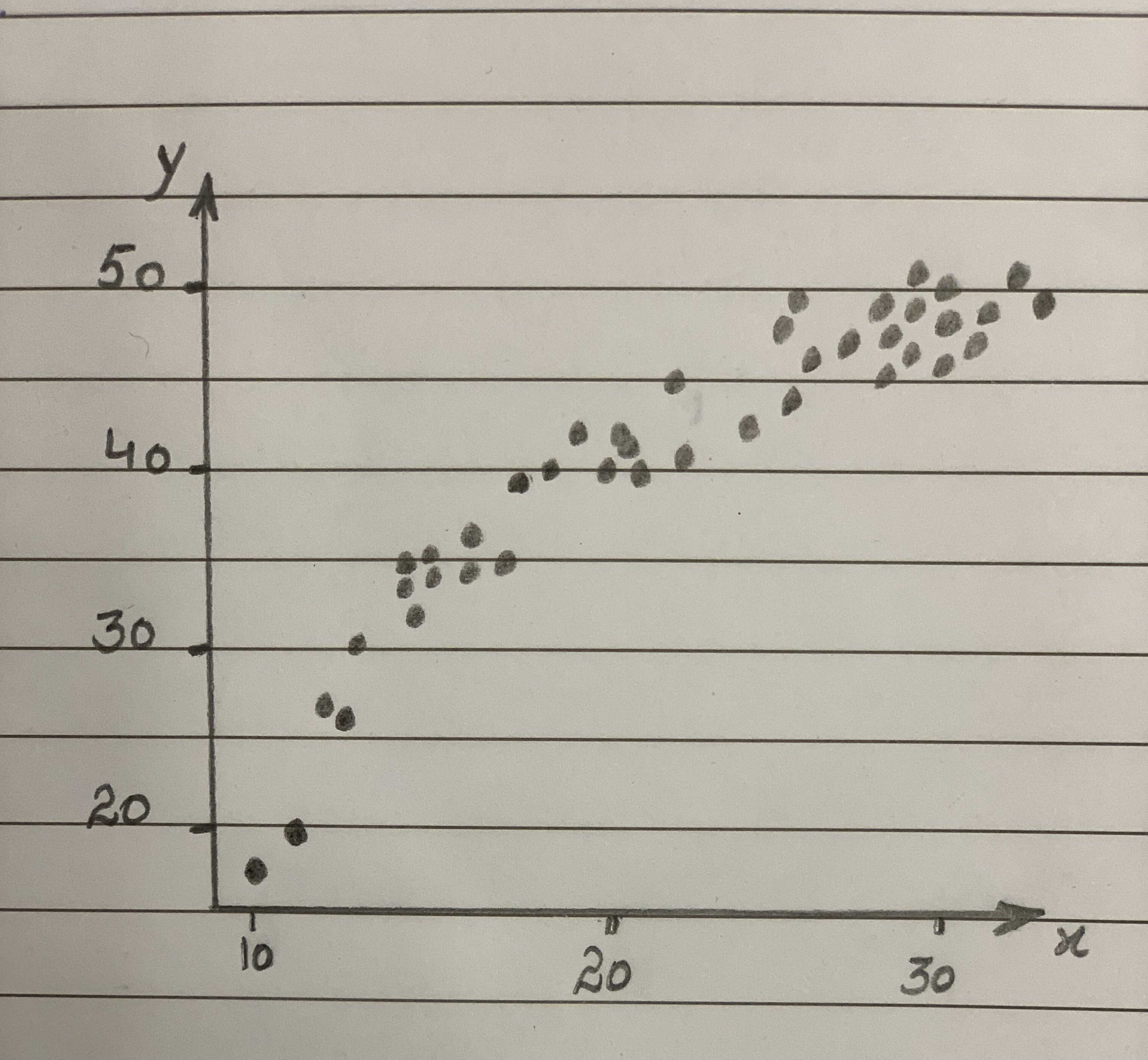2020-11-24
The provided scatter plot shows a curvature which indicates that Y values are increasing with the decrease of X values.
a. The points on y-axis indicate that power on y should be increased. There are many possible ways to straighten the scatter plot. The transformations that should be used to straighten the scatter plot is by increasing the power on Y. So, the transformed variables can be shown as y, $$y^(1.5), y^2, y^(2.5) and y^3$$.
Step 2
b. The mentioned scatter plot shows that there is a negative curvilinear relationship between the variables x and y.
The arrow on the x-axis points to the left indicates that the power on x should be decreased. The transformations that should be used to straighten the scatter plot is to decrease power on X. So, the transformed variables can be shown as,
$$x,sqrtx,1/sqrtx,1/x,In (x)$$

### Relevant QuestionsLily wants to define a transformation (or series of transformations) using only rotations, reflections or translations that takes Figure A to Figure B.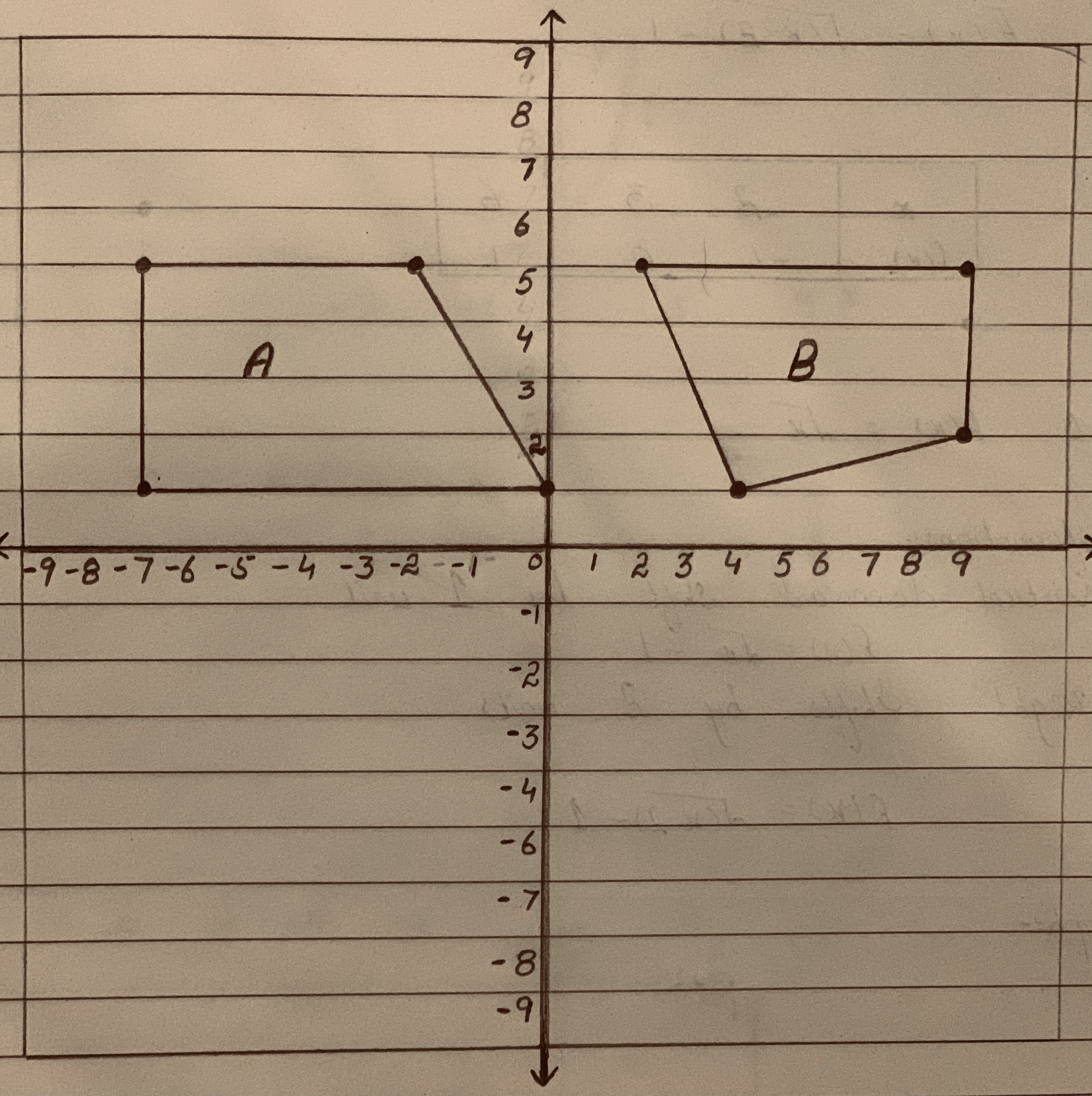Which statement about the transformation that Lily wants to define is true?
A. It can be defined with two reflections.
B.It can be defined with one rotation and one translation.
C. It cannot be defined because Figure A and Figure B are not congruent.
D.It cannot be defined because the longest side of Figure B is on the bottom.a. In using the method of transformations, we should only transform the predictor variable to straighten a scatterplot.
b. In using the method of transformations, a transformation of the predictor variable will change the conditional distribution of the response variable.
c. It is not always possible to fnd a power transformation of the response variable or the predictor variable (or both) that will straighten the scatterplot.Functions f and g are graphed in the same rectangular coordinate system (see attached herewith). If g is obtained from f through a sequence of transformations, find an equation for g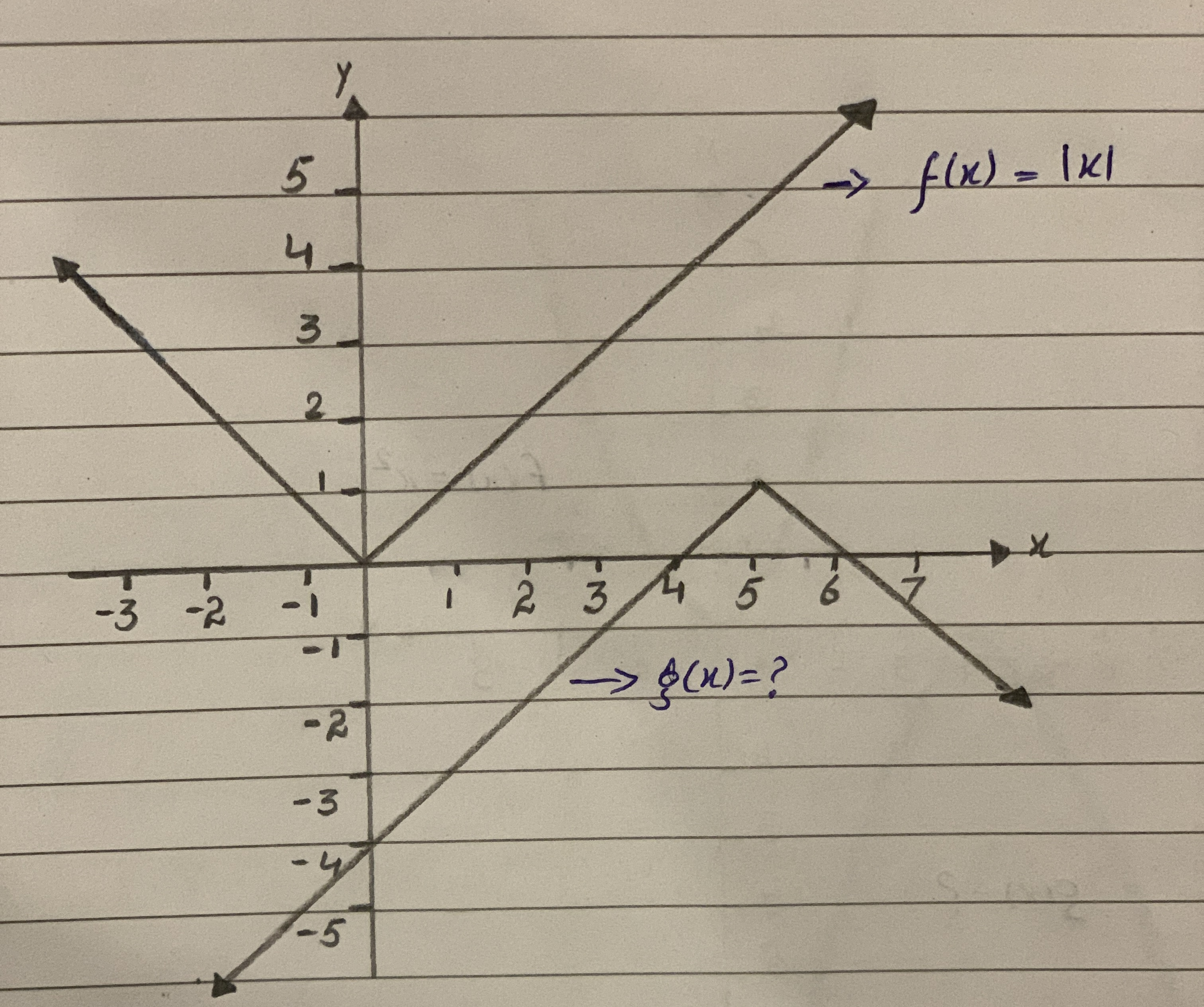Your friend attempted to describe the transformations applied to the graph of $$y=sinx$$ to give the equation $$f(x)=1/2sin(-1/3(x+30))+1$$.
They think the following transformations have been applied. Which transformations have been identified correctly, and which have not? Justify your answer.
a) f(x) has been reflected vertically.
b) f(x) has been stretched vertically by a factor of 2.
c) f(x) has been stretched horizontally by a factor of 3.
d) f(x) has a phase shift left 30 degrees.
e) f(x) has been translated up 1 unit.Predicting Land Value Both figures concern the assessed value of land (with homes on the land), and both use the same data set. (a). Which do you think has a stronger relationship with value of the land-the number of acres of land or the number of rooms n the homes? Why? b. Il you were trying to predict the value of a parcel of land in e arca (on which there is a home), would you be able to te a better prediction by knowing the acreage or the num- ber of rooms in the house? Explain. (Source: Minitab File, Student 12. "Assess.")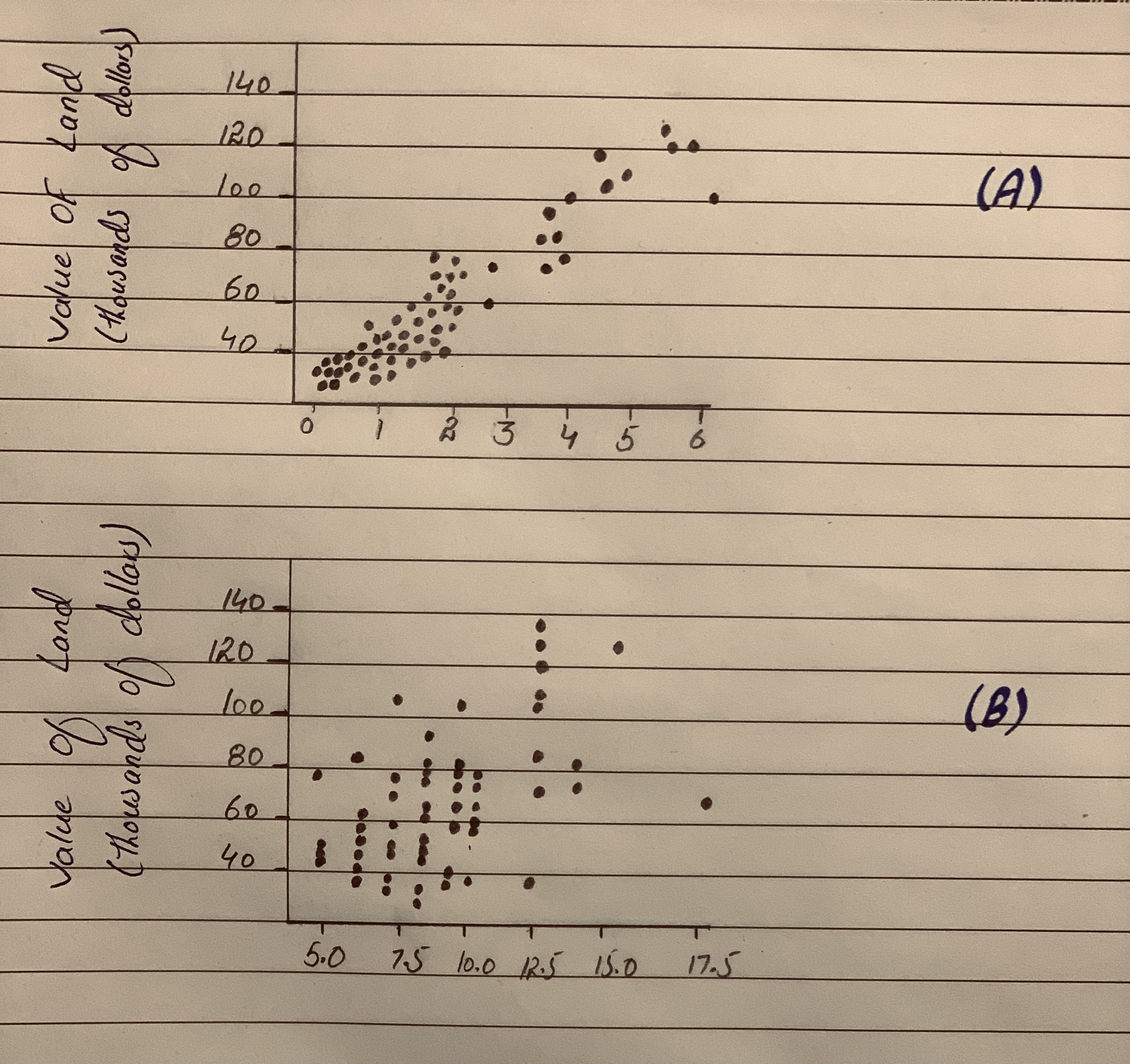Create a new function in the form $$y = a(x- h)^2 + k$$ by performing the following transformations on $$f (x) = x^2$$
Give the coordinates of the vertex for the new parabola.
h(x) is f (x) shifted right 3 units, stretched by a factor of 9, and shifted up by 7 units. h(x) = ?
Edit Coordinates of the vertex for the new parabola are:
x=?
y =?Create a new function in the form $$y = a(x-h)^2 + k$$ by performing the following transformations on $$f (x) = x^2$$.
Give the coordinates of the vertex for the new parabola.
g(x) is f (x) shifted right 7 units, stretched by a factor of 9, and then shifted down by 3 units. g(x) = ?
Coordinates of the vertex for the new parabola are:
x=?
y=?List a sequence of transformations, in words, to convert the graph of $$f(x)=x^2$$ into the graph of $$g(x)=-6(x-2)^2+5$$.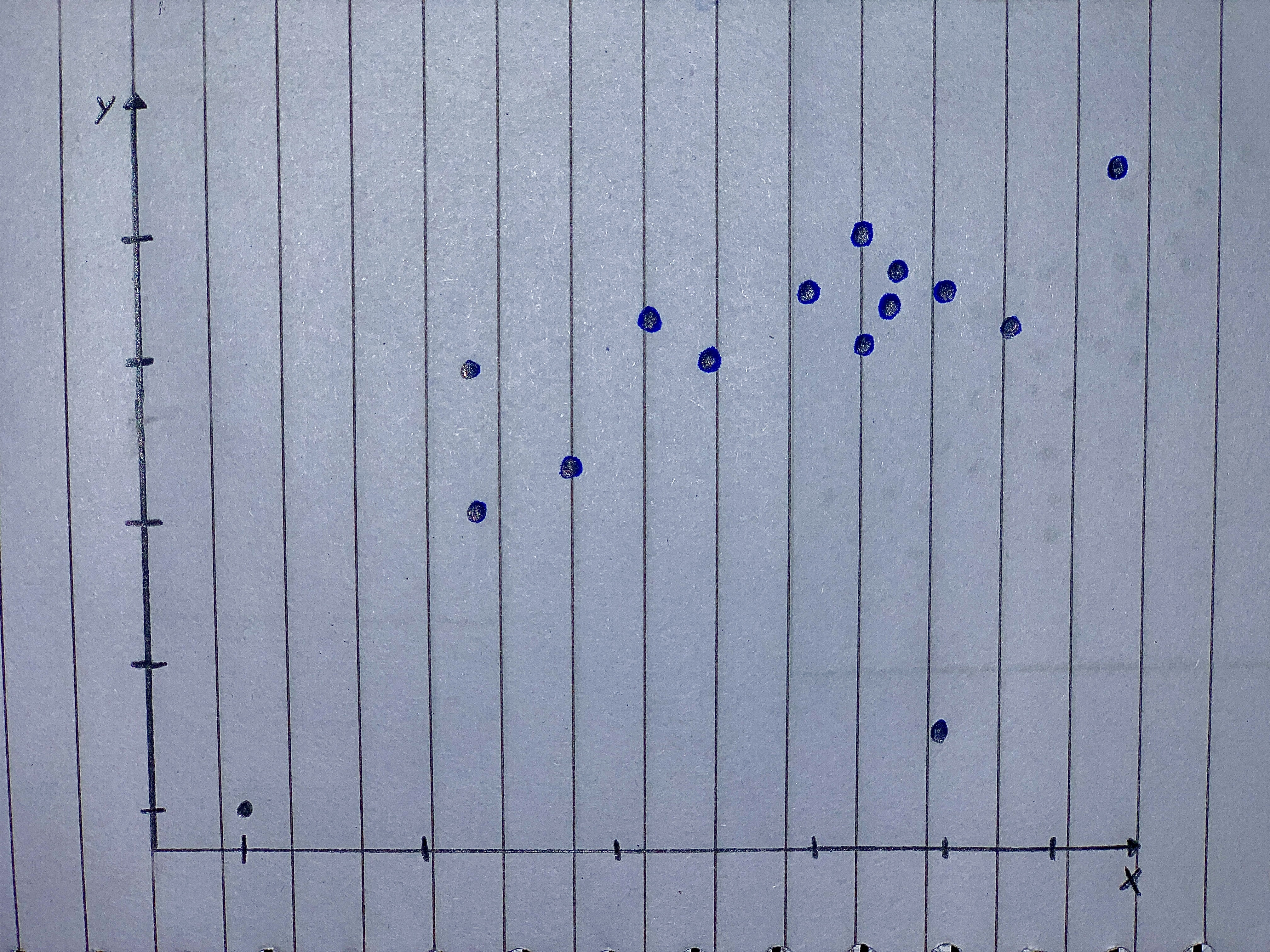Determine the transformations of the function f(x) to get the function $$g(x) =1/2f(5(x−3))−12$$ (The transformations happen in c, b, a, d order)